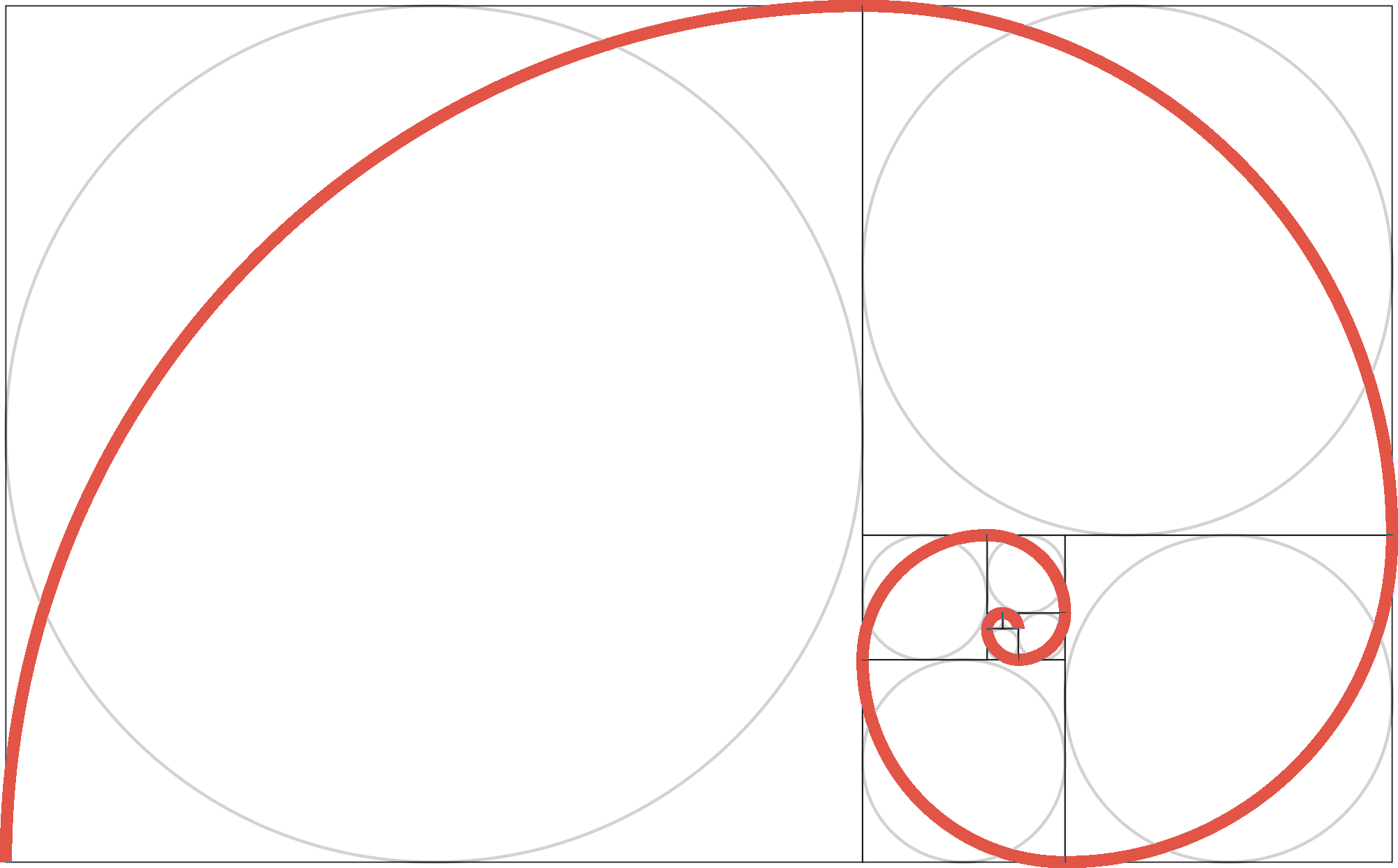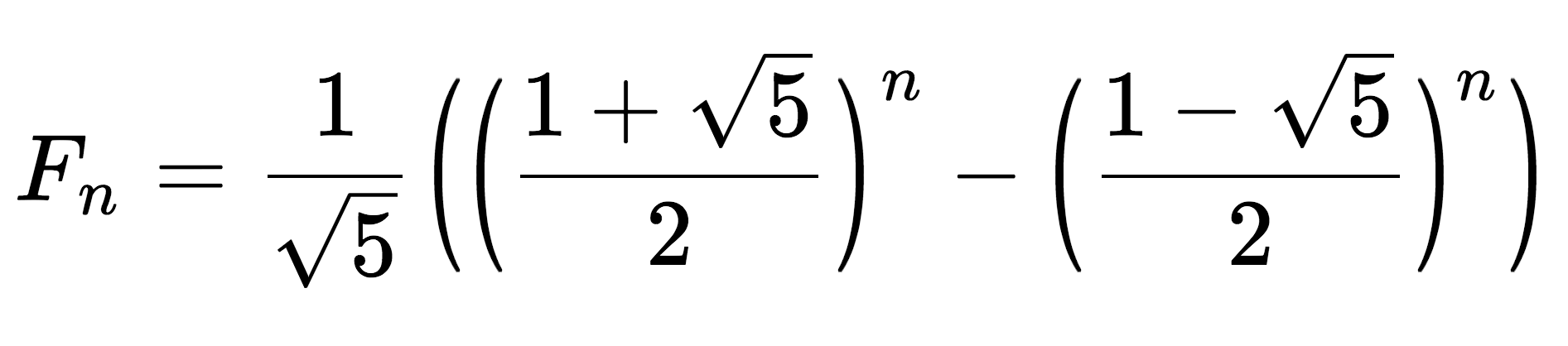# Fibonacci Sequence Calculator

Calculate the nth term in the Fibonacci sequence by entering the index n that you want to find.

## Fibonacci Number:

Fn =

Learn how we calculated this below

## What is the Fibonacci Sequence?

The Fibonacci sequence is a series of numbers where each number is equal to the sum of the previous two numbers in the sequence. Each number in the sequence is denoted as Fn, where n is the index of the number in the sequence.

The Fibonacci sequence starts with F0 and F1 equalling 0 and 1, respectively.

The Fibonacci sequence is often represented as a spiral, which is formed when creating squares with the width of each number in the sequence.## How to Calculate a Term in the Fibonacci Sequence

Because each term in the Fibonacci sequence is equal to the sum of the two previous terms, to solve for any term, it is required to know the two previous terms.

### Formula to Solve the Nth Fibonacci Term

The equation to solve for any term in the sequence is:

Fn = Fn-1 + Fn-2

Thus, the Fibonacci term in the nth position is equal to the term in the nth minus 1 position plus the term in the nth minus 2 position.

### Binet’s Formula

Named after French mathematician Jacques Philippe Marie Binet, Binet’s formula defines the equation to calculate the nth term in the Fibonacci sequence without using the recursive formula shown above.

Based on the golden ratio, Binet’s formula can be represented in the following form:

Fn = 1 / √5((1 + √5 / 2)n – (1 – √5 / 2)n)

Thus, Binet’s formula states that the nth term in the Fibonacci sequence is equal to 1 divided by the square root of 5, times 1 plus the square root of 5 divided by 2 to the nth power, minus 1 minus the square root of 5 divided by 2 to the nth power.

Binet’s formula above uses the golden ratio 1 + √5 / 2, which can also be represented as φ.## First 100 Numbers in the Fibonacci Sequence

The table below shows the first 100 numbers in the Fibonacci sequence.

First 100 numbers in the Fibonacci sequence.
n Fibonacci Number
0 0
1 1
2 1
3 2
4 3
5 5
6 8
7 13
8 21
9 34
10 55
11 89
12 144
13 233
14 377
15 610
16 987
17 1,597
18 2,584
19 4,181
20 6,765
21 10,946
22 17,711
23 28,657
24 46,368
25 75,025
26 121,393
27 196,418
28 317,811
29 514,229
30 832,040
31 1,346,269
32 2,178,309
33 3,524,578
34 5,702,887
35 9,227,465
36 14,930,352
37 24,157,817
38 39,088,169
39 63,245,986
40 102,334,155
41 165,580,141
42 267,914,296
43 433,494,437
44 701,408,733
45 1,134,903,170
46 1,836,311,903
47 2,971,215,073
48 4,807,526,976
49 7,778,742,049
50 12,586,269,025
51 20,365,011,074
52 32,951,280,099
53 53,316,291,173
54 86,267,571,272
55 139,583,862,445
56 225,851,433,717
57 365,435,296,162
58 591,286,729,879
59 956,722,026,041
60 1,548,008,755,920
61 2,504,730,781,961
62 4,052,739,537,881
63 6,557,470,319,842
64 10,610,209,857,723
65 17,167,680,177,565
66 27,777,890,035,288
67 44,945,570,212,853
68 72,723,460,248,141
69 117,669,030,460,994
70 190,392,490,709,135
71 308,061,521,170,129
72 498,454,011,879,264
73 806,515,533,049,393
74 1,304,969,544,928,657
75 2,111,485,077,978,050
76 3,416,454,622,906,707
77 5,527,939,700,884,757
78 8,944,394,323,791,464
79 14,472,334,024,676,221
80 23,416,728,348,467,685
81 37,889,062,373,143,906
82 61,305,790,721,611,591
83 99,194,853,094,755,497
84 160,500,643,816,367,088
85 259,695,496,911,122,585
86 420,196,140,727,489,673
87 679,891,637,638,612,258
88 1,100,087,778,366,101,931
89 1,779,979,416,004,714,189
90 2,880,067,194,370,816,120
91 4,660,046,610,375,530,309
92 7,540,113,804,746,346,429
93 12,200,160,415,121,876,738
94 19,740,274,219,868,223,167
95 31,940,434,634,990,099,905
96 51,680,708,854,858,323,072
97 83,621,143,489,848,422,977
98 135,301,852,344,706,746,049
99 218,922,995,834,555,169,026
100 354,224,848,179,261,915,075